# LBP原理与OpenCV实现

## LBP简介

LBP(Local Binary Pattern)算法是一种描述图像特征像素点与各个像素点之间的灰度关系的局部特征的非参数算法，同时也是一张高效的纹理描述算法，纹理是物体表面的自然特性，它描述图像像素点与图像领域之间的灰度空间的分布关系，不会因为光照强弱而改变图像的视觉变化。LBP算法首次提出于1994年，主要是使用8邻域位置的局部关系，具有灰度不变性；随后在2002年提出了其改进版《Multiresolution gray-scale and rotation invariant texture classification with local binary patterns》 Timo Ojala, Matti PietikaÈ inen,2002，主要引入了圆形模式、旋转不变性、和等价模式，所以在下面我们将按照如下顺序介绍LBP特征：灰度不变性的基本LBP，灰度不变性的圆形LBP，旋转不变性的LBP，等价LBP，最后再继续进行我们上一次HOG+KNN的实验，用LBP特征提取+KNN算法实现手写数字识别问题。

## 灰度不变性基本LBP算法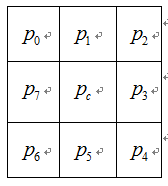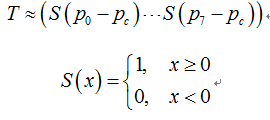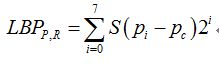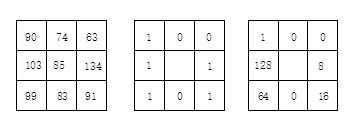## 灰度不变性圆形LBP算法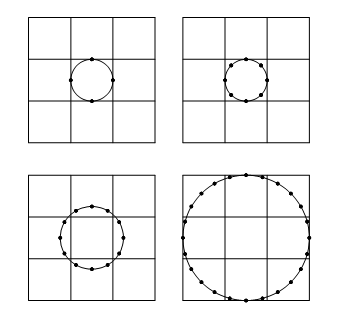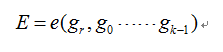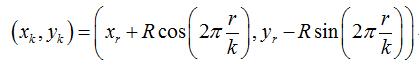## 旋转不变性LBP算法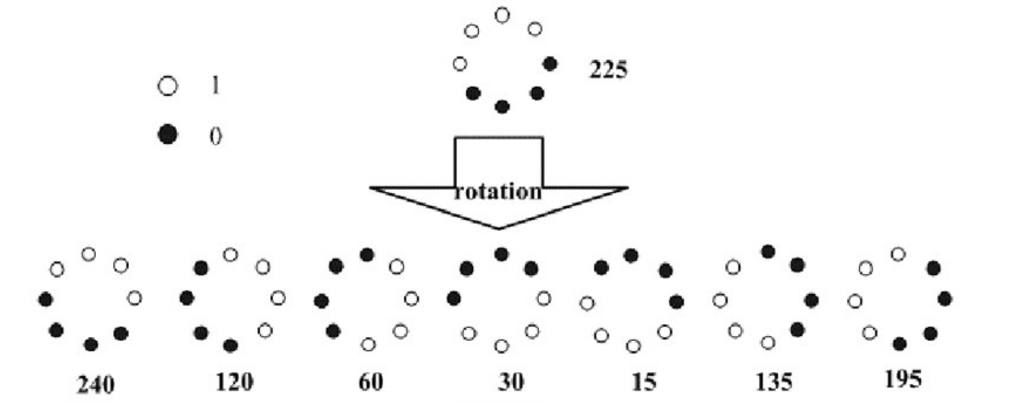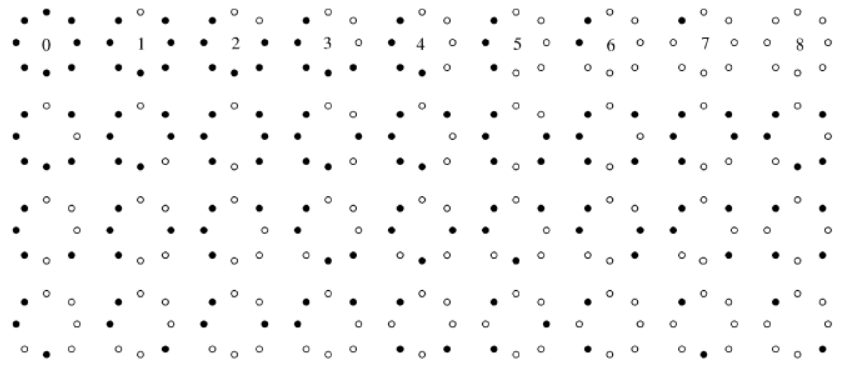#include <iostream>
#include <string>
#include <math.h>
using namespace std;

int ConversiontoDecimal(string input)
{
int Decimal=0;
for (int i=0;i<8;i++)
{
int n = input[i]-'0';
Decimal = Decimal+n*pow(2.0,i);
}
return Decimal;
}
string ConversiontoBinary(int n)
{
string s;
for(int a = n; a ;a = a/2)
s=s+(a%2?'1':'0');
reverse(s.begin(),s.end());
for (int i = s.size();i<=7;i++)
s = "0"+s;
return s;
}
int FindMin(string input)
{
int MinValue = 257;
for(int i =0;i<8;i++)
{
string str1 =input.substr(0,7);
string str2 = input.substr(7,1);
input = str2+str1;
int Decimal_Value = ConversiontoDecimal(input);
//cout<<Decimal_Value<<endl;
if(Decimal_Value<MinValue)
MinValue = Decimal_Value;
}
return MinValue;
}
int main()
{
int Hash;
memset(Hash,0,sizeof(Hash));
for (int i =0;i<256;i++)
{
string Binary =ConversiontoBinary(i);
//cout<<Binary<<endl;
int Min_Value = FindMin(Binary);
if (Hash[Min_Value]==0)
Hash[Min_Value]=1;
}
int NumofModle =0;
for (int i =0;i<256;i++)
NumofModle +=Hash[i];
cout<<NumofModle<<endl;
}


## LBP +KNN实现手写数字识别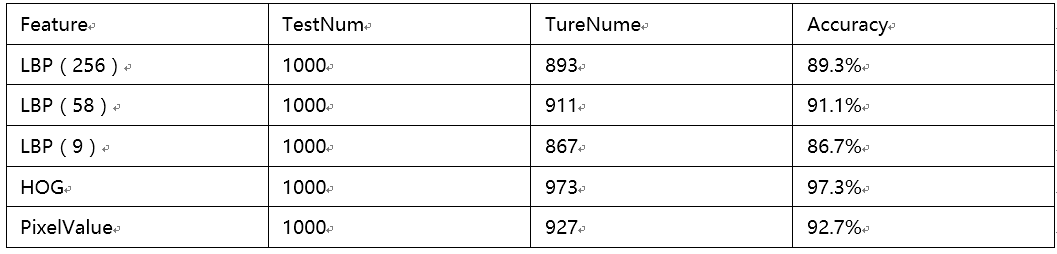PS：
1. 数据准备和KNN的部分在上一篇HOG特征中已提及，为节省篇幅不再重复
2. 整个代码有大概500行左右，为了节省篇幅，不贴代码啦，如果大家感兴趣点击这里查看。

08-111万+06-28125
04-242527
08-11209
02-215万+
01-304万+
05-234635
01-035468
©️2020 CSDN 皮肤主题: Age of Ai 设计师:meimeiellie